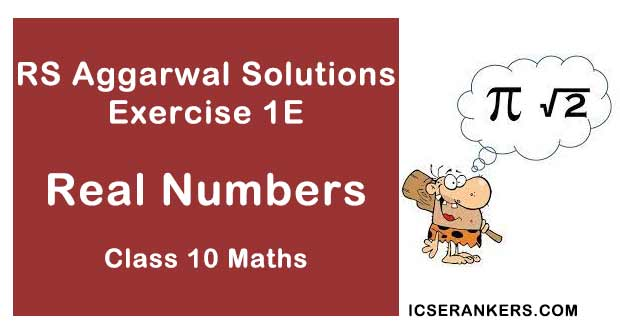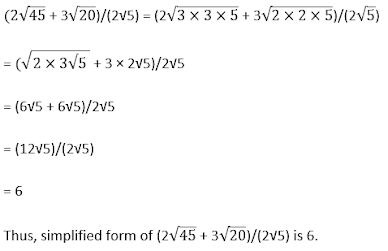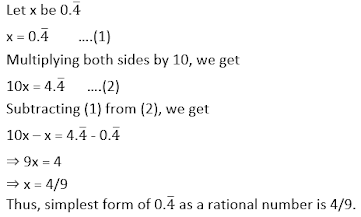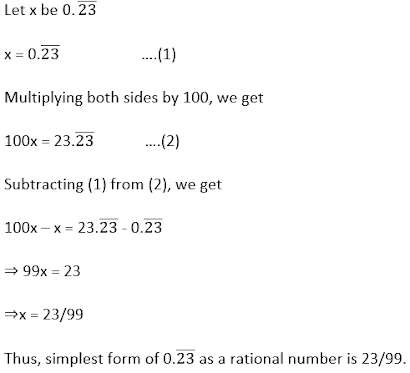# RS Aggarwal Solutions Chapter 1 Real Numbers Exercise - 1E Class 10 MathsChapter Name RS Aggarwal Chapter 1 Real Numbers Solutions Book Name RS Aggarwal Mathematics for Class 10 Other Exercises Exercise 1AExercise 1BExercise 1CExercise 1D Related Study NCERT Solutions for Class 10 Maths

### Exercise 1E Solutions

1. What do you mean by Euclid’s division algorithm.

Solution

Euclid’s division lemma, states that for any two positive integers a and b, there exist unique whole numbers q and r, such that

a = b × q + r where 0 ≤ r ˂ b

2State fundamental theorem of arithmetic?

Solution

The fundamental theorem of arithmetic, states that every integer greater than 1 either is prime itself or is the product of prime numbers, and this product is unique.

3. Express 360 as product of its prime factors

Solution

Prime factorization:

360 = 23 × 32 × 5

4. If a and b are two prime numbers then find the HCF (a, b)

Solution

Prime factorization:

a = a

b = b

HCF = product of smallest power of each common factor in the numbers = 1

Thus, HCF(a, b) = 1

5. If a and b are two prime numbers then find the HCF(a, b)

Solution

Prime factorization:

a = a

b = b

LCM = product of greatest power of each prime factor involved in the numbers = a × b

Thus, LCM (a, b) = ab

6. The product of two numbers is 1050 and their HCF is 25. Find their LCM.

Solution

HCF of two numbers = 25

Product of two numbers = 1050

Let their LCM be x.

Using the formula, Product of two numbers = HCF × LCM

We conclude that,

1050 = 25 × x

⇒ x = 1050/25

⇒ x = 42

Hence, their LCM is 42.

7. What is a composite number?

Solution

A composite number is a positive integer which is not prime (i.e. which has factors other than 1 and itself).

8. If a and b are relatively prime then what is their HCF?

Solution

If two numbers are relatively prime then their greatest common factor will be 1.

Thus, HCF (a, b) = 1.

9. If the rational number a/b has a terminating decimal expansion, what is the condition to be satisfied by b?

Solution

Let x be a rational number whose decimal expansion terminates.

Then, we can express x in the form a/b, where a and b are coprime, and prime factorization of b is of the form (2m × 5n), where m and n are non-negative integers.

10. Find the simplest form of (2√45 + 3√20)/2√5

Solution11. Write the decimal expansion of 73/(24 × 53)

Solution

Decimal expansion:

73/(24 × 53) = (73 × 5)/(24 × 54)

= 365/(2 × 5)4

= 365/(10)4

= 365/10000

= 0.0365

Thus, the decimal expansion of 73/(24 × 53) is 0.0365.

12. Show that there is no value of n for which (2n × 5n) ends in 5.

Solution

We can write:

(2n × 5n) = (2 × 5)n

= 10n

For any value of n, we get 0 in the end.

Thus, there is no value of n for which (2n × 5n) ends in 5.

13. Is it possible to have two numbers whose HCF if 25 and LCM is 520?

Solution

No, it is not possible to have two numbers whose HCF is 25 and LCM is 520.

Since, HCF must be a factor of LCM, but 25 is not a factor of 520.

14. Give an example of two irrationals whose sum is rational.

Solution

Let the two irrationals be 4 - √5 and 4 + √5

(4 - √5) + (4 + √5) = 8

Thus, sum (i.e., 8) is a rational number.

15. Give an example of two irrationals whose product is rational.

Solution

Let the two irrationals be 4 √5 and 3 √5

(4 √5) × (3 √5) = 60

Thus, product (i.e., 60) is a rational number.

16. If a and b are relatively prime, what is their LCM?

Solution

If two numbers are relatively prime then their greatest common factor will be 1.

∴ HCF (a, b) = 1

Using the formula, Product of two numbers = HCF × LCM

we conclude that,

a × b = 1 × LCM

∴ LCM = ab

Thus, LCM (a, b) is ab.

17. The LCM of two numbers is 1200, show that the HCF of these numbers cannot be 500. Why?

Solution

If the LCM of two numbers is 1200 then, it is not possible to have their HCF equals to 500. Since, HCF must be a factor of LCM, but 500 is not a factor of 1200.

18. Express 0.4 as a rational number simplest form.

Solution19. Express 0.23 as a rational number in simplest form.

Solution20. Explain why 0.15015001500015… is an irrational form.

Solution

Irrational numbers are non-terminating non-recurring decimals.

Thus, 0.15015001500015…. is an irrational number.

21. Show that √2/3 is irrational.

Solution

Let √2/3 is a rational number.

∴ √2/3 where p and q are some integers and HCF (p, q) = 1 ...(1)

⇒ √2q = 3p

⇒ (√2q)2 = (3p)2

⇒ 2q2 = 9p2

⇒ p2 is divisible by 2

⇒ p is divisible by 2 ...(2)

Let p = 2m, where m is some integer.

∴ √2q = 3p

⇒ √2q = 3(2m)

⇒ (√2q)2 = [3(2m) ]2

⇒ 2q2 = 4(9p2)

⇒ q2 = 2 (9p2

⇒ q2 is divisible by 2

⇒ q is divisible by 2 …(3)

From (2) and (3), 2 is a common factor of both p and q, which contradicts (1).

Hence, our assumption is wrong.

Thus, √2/3 is irrational.

22. Write a rational number between √3 and 2

Solution

Since, √3 = 1.732….

So, we may take 1.8 as the required rational number between √3 and 2.

Thus, the required rational number is 1.8.

23. Explain why 3.1416 is a rational number?

Solution

Since, 3.1416 is a non-terminating repeating decimal.

Hence, is a rational number.